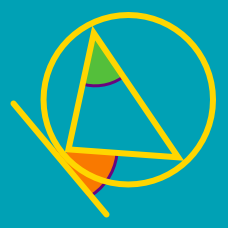Geometry

# Tangent and Secant Lines: Level 3 Challenges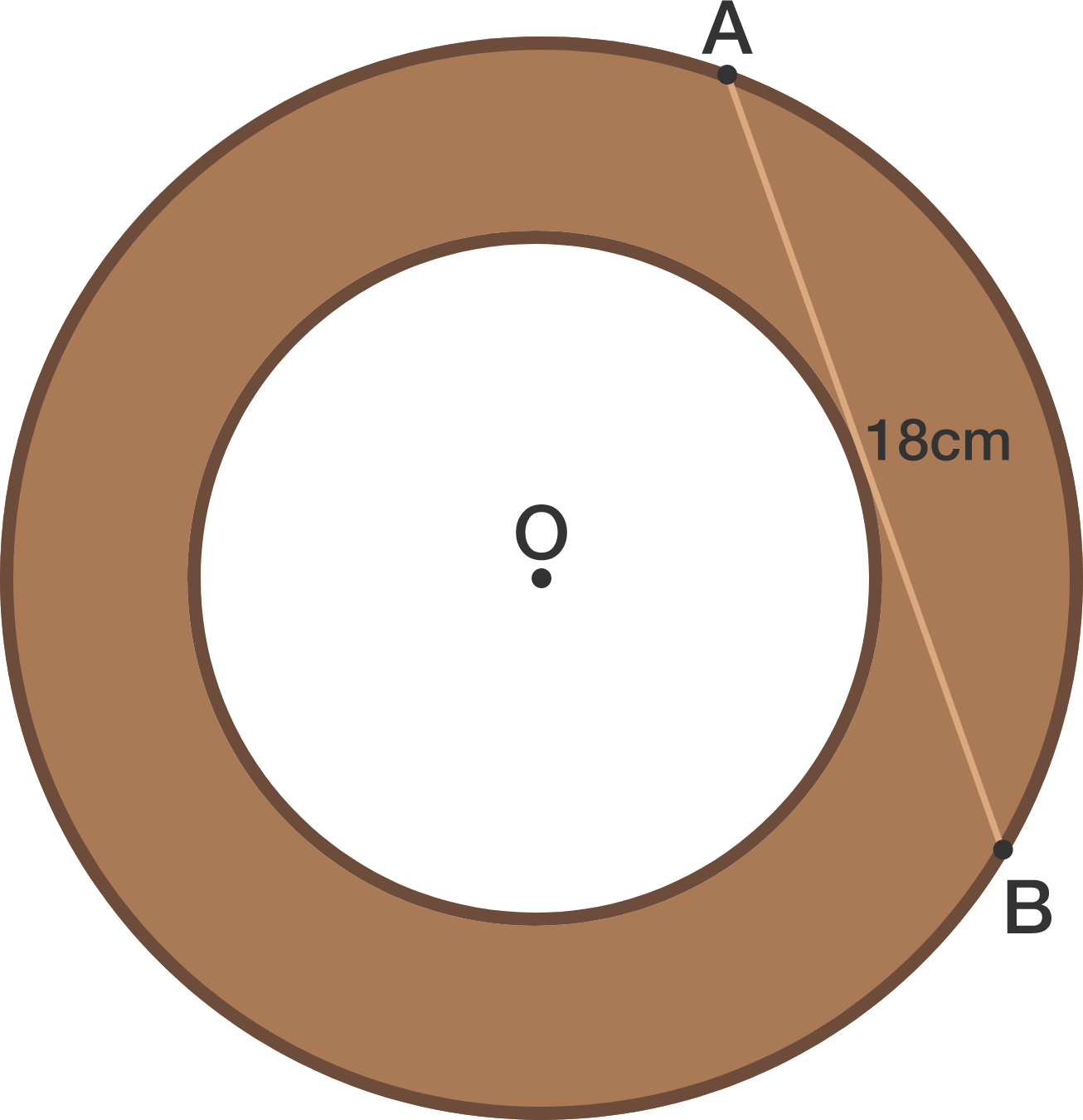$O$ is the center of the circle. If $AB = 18$ cm, then the area of the brown part is $x \pi$. What is $x$?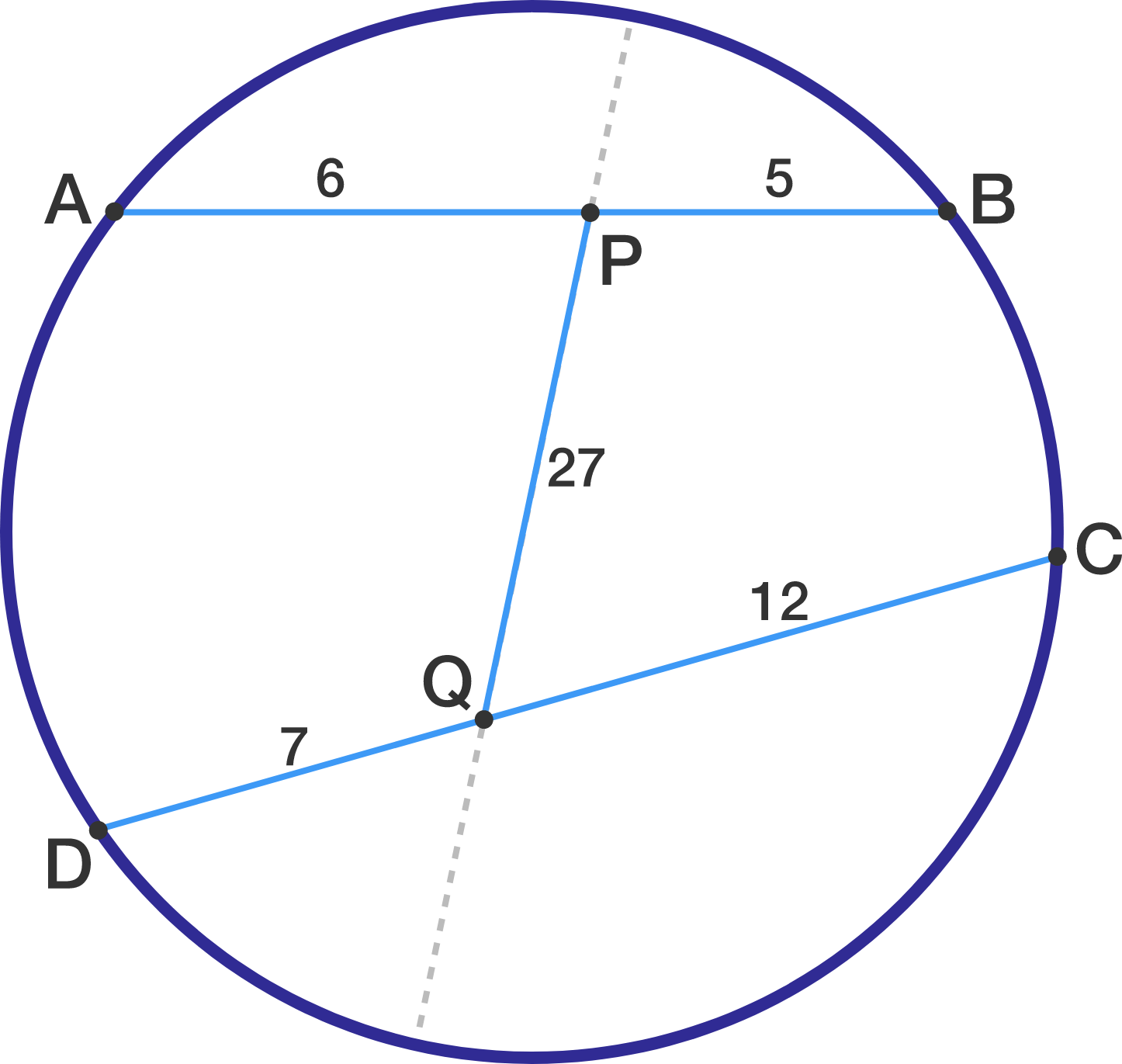$ABCD$ is a cyclic quadrilateral with $\displaystyle \overline{AB}=11$ and $\displaystyle \overline{CD}=19$. $P$ and $Q$ are points on $\overline{AB}$ and $\overline{CD}$, respectively, such that $\displaystyle \overline{AP}=6$, $\displaystyle \overline{DQ}=7$, and $\displaystyle \overline{PQ}=27.$ Determine the length of the line segment formed when $\displaystyle \overline{PQ}$ is extended from both sides until it reaches the circle.

Note: The image is not drawn to scale.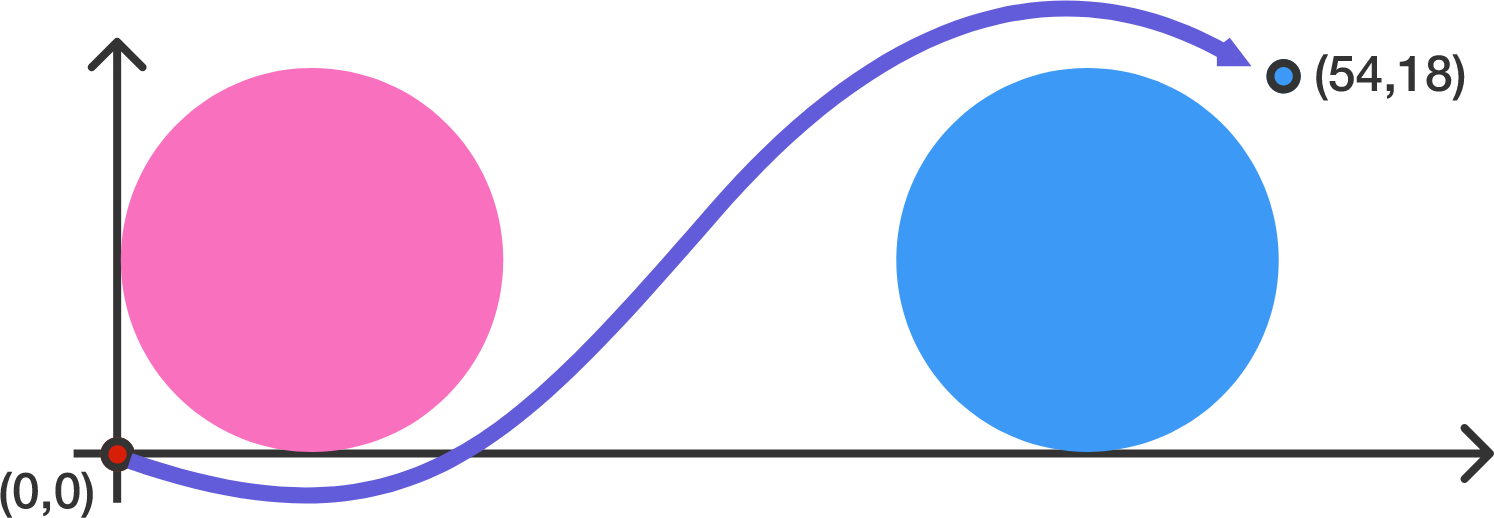You are currently located on point $(0,0)$ and you want to get on point $(54,18)$. However, there are some annoying circular objects in the way! They are defined by $x^2+y^2-18x-18y+81=0$ $x^2+y^2-90x-18y+2025=0$

If you cannot walk through these annoying circular objects, then the shortest possible path possible to point $(54,18)$ can be expressed as $a+b\sqrt{c}+d\pi$ for positive integers $a,b,c,d$ with $c$ square-free. What is $a+b+c+d$?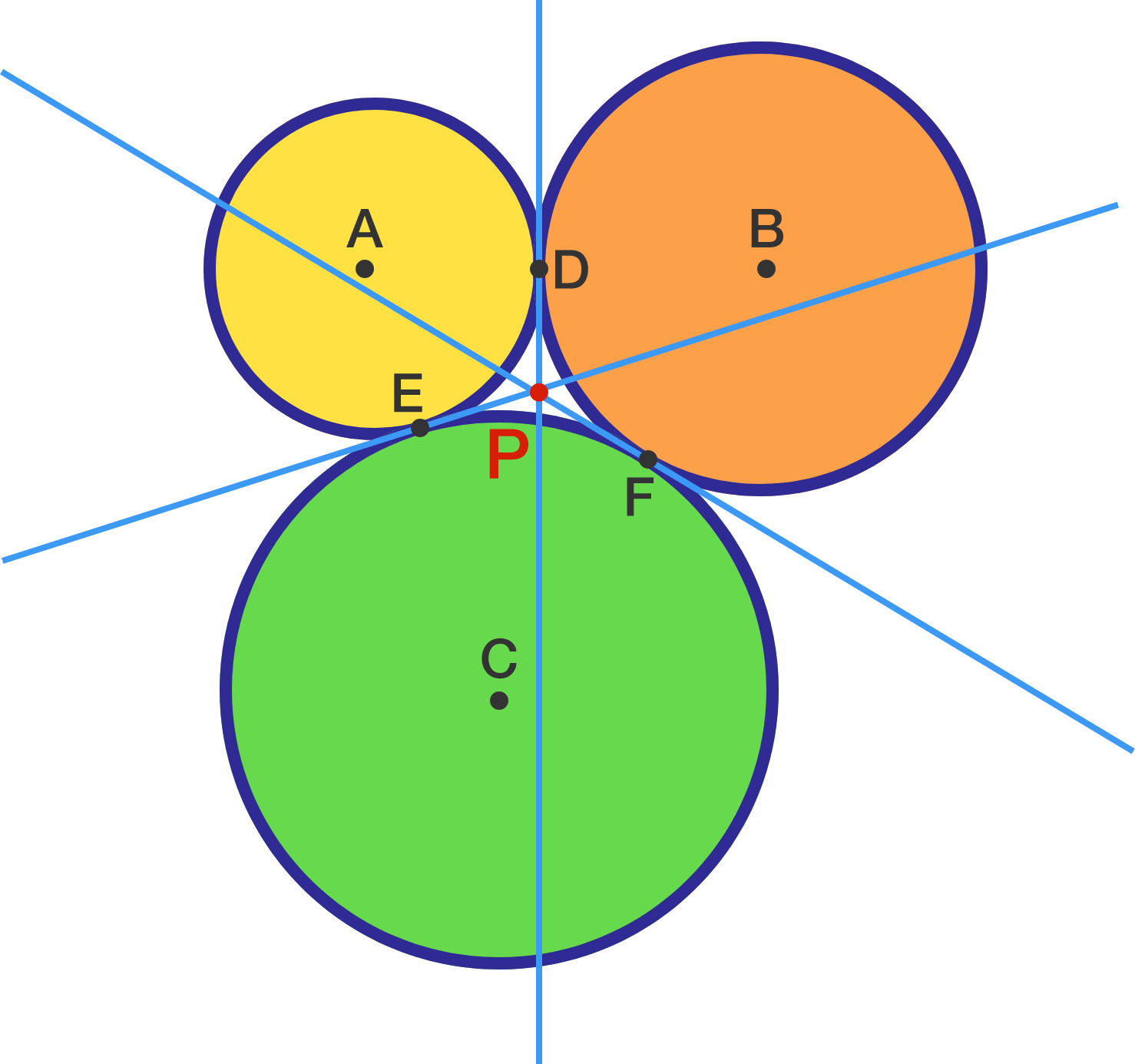Circles of radii 3,4, and 5 units are externally tangent. The lines which form the 3 common external tangent intersect at $P,$ which is equidistant from the 3 points of tangency. Find this distance (from $P$ to any point of tangency)?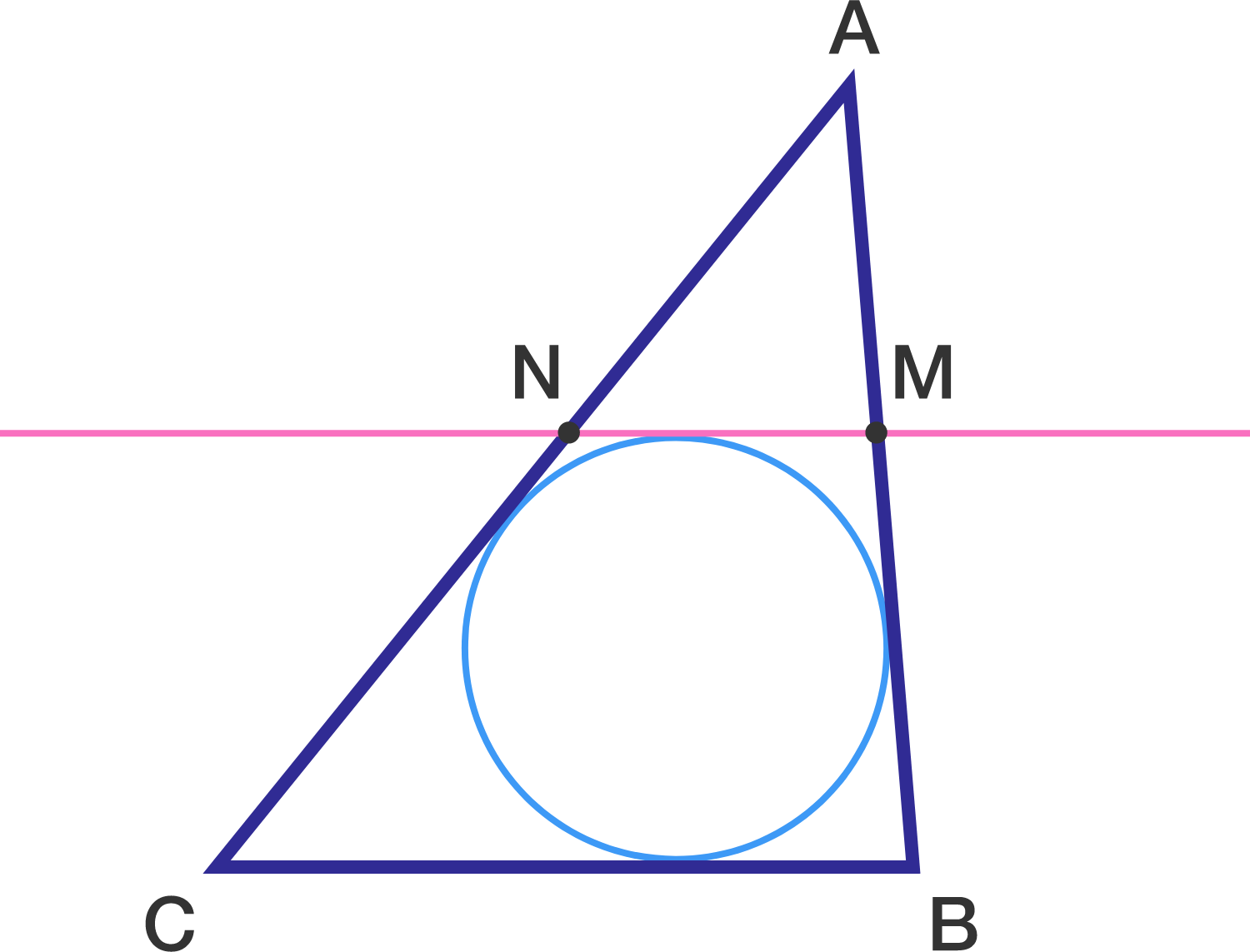In $\triangle ABC,$ $AB=6$ , $BC = 4$ and $AC=8.$ A segment parallel to $\overline{BC}$ and tangent to the incircle of $\triangle ABC$ intersects $\overline{AB}$ at $M$ and $\overline{AC}$ at $N$.

If $MN=\dfrac{a}{b}$, where $a$ and $b$ are co-prime positive integers, what is the value of $a+b$ ?

×